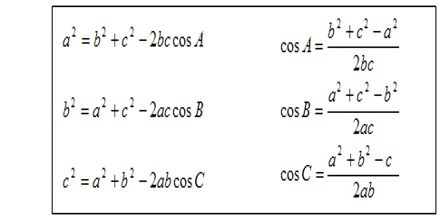Mathematic

# The Cosine RuleGeneral objective of this lecture is to present on the Cosine Rule. Sometimes the sine rule is not enough to help us solve for a non-right angled triangle. We can use the cosine formula to find the length of a side or size of an angle. For a triangle with sides a,b and c and angles A, B and C the cosine rule can be written as: a² = b² + c² – 2bc cos A. The Cosine Rule maybe used when: Two sides and an included angle are given and Three sides are given.